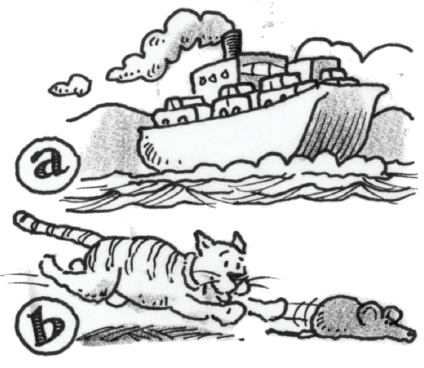### Home > APCALC > Chapter 4 > Lesson 4.2.2 > Problem4-58

4-58.

Compare how distance and velocity are related with these two scenarios:

1. A ferry crosses the bay so that its distance (in miles) from the dock at time $t$ is $d\left(t\right)=1- \cos\left(t\right)$. What is the velocity, $d ^\prime(t)$, at times $t = 1$, $π$, and $5$ hours? Explain what concepts of calculus you applied in order to solve this problem.

Explain WHY $d^\prime\left(t\right) = v\left(t\right)$. Describe what velocity looks like on a distance graph.

2. When a cat chases a mouse, the cat’s velocity, measured in feet per second, is $v(t) = 3t$. Sketch a graph and calculate the distance the cat ran in the first $5$ seconds. Explain what concepts of calculus you applied in order to solve this problem.

Describe what distance (or displacement) looks like on a velocity graph.

3. Both (a) and (b) involve distance and velocity. However, each required a different method or approach. Describe the relationship between distance and velocity, mentioning the derivative and area under a curve.